# Cement blocks

Each cement block weighs 7.9 kilograms. How much do 6 blocks weigh in total?

m =  47.4 kg

### Step-by-step explanation: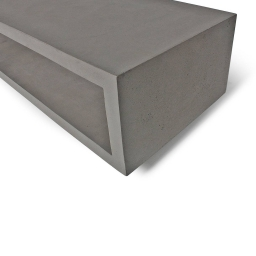Did you find an error or inaccuracy? Feel free to write us. Thank you!## Related math problems and questions:

• Colored blocksTucker and his classmates placed colored blocks on a scale during a science lab. The brown block weighed 8.94 pounds, and the red block weighed 1.87 pounds. How much more did the brown block weigh than the red block?
• Weigh in totalI put 3/5 kg of grapes into a box which is 1/4kg in weight. How many kilograms do the grapes and the box weigh in total?
• Water in body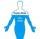Water in the human organism of all the compounds represented constitutes about 3/5 of the total weight. How many kilograms of water is in your body when you weigh 51 kg?
• Warehouses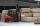To four warehouses is going cement in 25 kg bags. To first one third, to second quarter of the total. The third store got two thirds of the rest, and the last 310 tons came to fourth. How many cement is in all warehouses and how much got every one?
• Can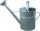Watering can full of water weighs 10 kg. Half-full can weighs 5.5 kg. How much weigh can?
• Freight train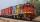Twenty-five identical freight wagons are connected behind the locomotive. The weight of the locomotive and all wagons is 1009000 kg. The locomotive weighs 84 tons. How many kilograms does one freight car weigh?
• Buns and toastsTwo buns weigh 10 grams more than two toasts. One Bun and two Toast weigh a total of 110 grams. How many grams weigh three toasts? How many grams does a Bun weigh?
• One kilogramThe apple weighs 125 grams and half apple. How many apples weigh 1 kilogram?
• Empty aquariumHow much does an empty aquarium weigh with dimensions: length = 40 cm, width = 30 cm, height = 20 cm, if 1 dm2 of glass weighs 300 g? Calculate its weight in kilograms.
• Two bricks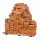One brick weighs 1kg and half a brick, how many weigh two bricks?
• Brick weightThe brick weighs 2 kg and a half bricks. How much does one brick weigh?
• Bag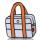Nelly found interesting point. An empty bag weighs 4 kg less than full. And empty bag is 5 times easier than full. How many kg weigh things in this bag?
• Train tons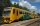The entire loaded train weighs 760 tons. It has a total of 30 wagons, each carrying 12 tons of grain and empty weighs 10 tons. What is the weight of the locomotive?
• Children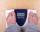Margaret and Zdena weigh the same and Petra 3 kg more. Together weigh 156 kg. How much do they weigh?
• Eight blocks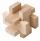Dana had the task to save the eight blocks of these rules: 1. Between two red cubes must be a different color. 2. Between two blue must be two different colors. 3. Between two green must be three different colors. 4. Between two yellow blocks must be four
• A bottleA bottle full of cola weighs 1,320 g. If we drink three-tenths of it, it will weigh 1,008g. How much does an empty bottle weigh?
• Dinesh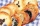Dinesh ate 3 cookies, each with mass 1.45 g. What was the total mass of the cookies that he ate?